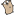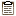## Go by Example 中文版: 函数

 函数 是 Go 的核心。我们将通过一些不同的例子来进行学习它。```package main ``` ```import "fmt" ``` 这里是一个函数，接受两个 `int` 并且以 `int` 返回它们的和 ```func plus(a int, b int) int { ``` Go 需要明确的 return，也就是说，它不会自动 return 最后一个表达式的值 ``` return a + b } ``` 当多个连续的参数为同样类型时， 可以仅声明最后一个参数的类型，忽略之前相同类型参数的类型声明。 ```func plusPlus(a, b, c int) int { return a + b + c } ``` ```func main() { ``` 通过 `函数名(参数列表)` 来调用函数， ``` res := plus(1, 2) fmt.Println("1+2 =", res) ``` ``` res = plusPlus(1, 2, 3) fmt.Println("1+2+3 =", res) } ```
 ```\$ go run functions.go 1+2 = 3 1+2+3 = 6 ``` Go 函数还有很多其他的特性。 其中一个就是多值返回，它也是我们接下来要接触的。